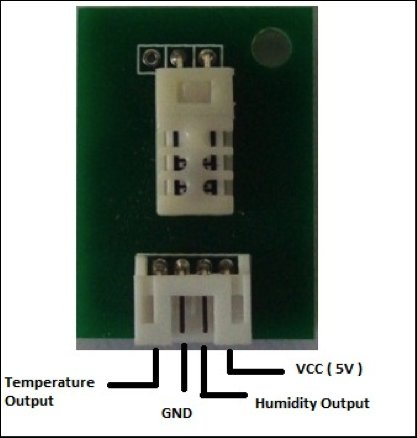# HSM-20G Interface with Arduino UnoThe HSM-20G is an analog humidity and temperature sensor that outputs analog voltage respects to relative humidity and temperature. However from this sensor relative humidity is found along with temperature. Relative humidity is the percentage of moistures of airs for a particular temperature .
Feature:
1. Storage range: -20°C to 70°C
2. Operating range: 0°C to 50°C## Application:

HSM-20g is considered as the most operating sensor compared to other similar products. This sensor is used in BTS (base transmission station) for weather forecasting. Also it is used in:
1. Air-conditioner
2. Automatic climate control system
3. Humidity data logger
4. Weather monitoring system

## Parts Needed:

1. Arduino uno (1pc)
2. HSM-20G (1pc)
3. Resistors- 100K, 10K
4. Capacitor- 47uF/10V

## Wiring:

Usually for arduino interfacing sensor data pins are directly feed to arduino I/O pin. However for this HSM-20G sensor a particular circuit connection needed to be followed for getting desired result.
1. Connects all resistors and capacitor

. Then Connect humidity output with arduino analog pin A0 and temperature outpin pin with arduino analog pin A1. In this article, I used A0 and A1 pins, but user can select any of analog pins for communication.
3. If circuit connection is set accurately then the hardware is ready, however we need to do arduino coding.

## Code:

Arduino Coding is done in Arduino IDE.

``````
void setup()
{
Serial.begin(9600); // Serial monitor baud rate
Serial.println("setup");
}
void loop()
{
// eqn
int voltage = ((humValue*5.0)/1023.0); // convert analog value to voltage
//equation for humidity
int humidity= (3.71*pow(voltage,3))-(20.65*pow(voltage,2))+(64.81*voltage)-27.44;

Serial.print("Humidity: "+humidity);
Serial.println(" %");
delay(1000);
int voltage_temp = ((tempValue*5.0)/1023.0); // convert analog value to voltage
//equation for temperature
int temperature=(5.26*pow(voltage_temp,3))-(27.34*pow(voltage_temp,2))+(68.87*voltage_temp)-17.81;
Serial.print("Temperature: "+temperature);
Serial.println(" C");
}
``````

## Discussion:

The Arduino board has an analog-to-digital converter from A0-A5 pin that reads this changing voltage and converts it to a number between 0 and 1023. So from A0 and A1 pin at first the analog values are converted to voltage. Since the sensor uses a specific connection for wiring, for getting desired value for humidity and temperature two equations are derived from the graphs given in datasheet.

Read more: HSM-20G Interface with Arduino Uno